2018-12-14 20:41:49 zijin0802034 阅读数 10186
• ###### 卷积神经网络与人脸识别系统实战视频教程

卷积神经网络与人脸识别系统实战视频培训课程概况：本教程从零开始讲解人工神经网络，卷积神经网络等基础内容，然后讲解基于python如何构建一个深度学习模型，后基于CNN，利用keras构建网络结构打造一个人脸识别系统。

2558 人正在学习 去看看 王而川

# 简介# Contrastive Loss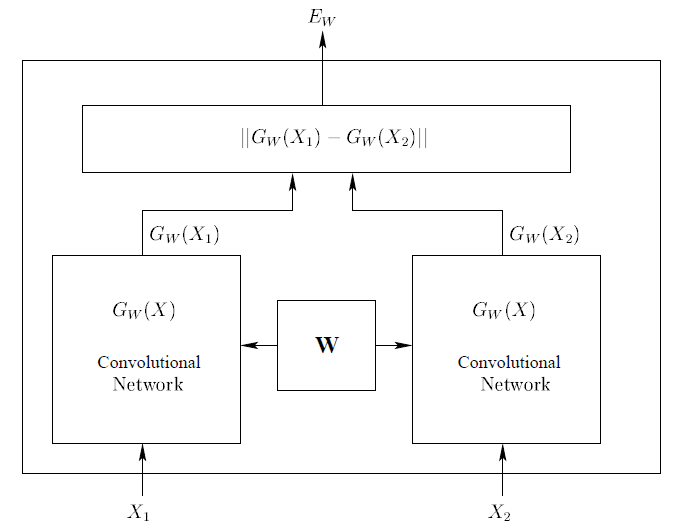Contrastive loss 定义如下：
$l(W)=∑_{i=1}^P L(W,(Y,X_1,X_2 )^i)$

$L(W,(Y,X_1,X_2 )^i )=(1-Y) L_G (E_W (X_1,X_2 )^i )+YL_I (E_W (X_1,X_2 )^i)$

$L(W,(Y,X_1,X_2 )^i )=(1-Y) E_W (X_1,X_2 )^i-Y(E_W (X_1,X_2 )^i))$

# Triplet Loss

FaceNet  文中提出了Triplet Loss，与Contrastive loss的输入二元组不同的是，Triplet输入时人脸三元组。随机从某个人照片中选择一张，叫做Anchor；再从这个人照片中选择另一个照片，叫做Positive；最后随机选择一张其他人的照片，叫做Negative， 这样就构成了图像的三元组。图5就是三元组的一个例子。$L= ∑_i^N [∥f(x_i^a )-f(x_i^p )∥_2^2-∥f(x_i^a )-f(x_i^n )∥_2^2+α]_+$

# Center Loss

$L_C= \frac{1}{2} ∑_{i=1}^m (∥x_i-c_{y_i}∥)_2^2$

$C_{yi} \in R^d$表示第$Y_i$ 类的特征中心。Center loss目标是让每类的特征尽可能集中，让每类在特征空间内所占的范围尽可能小，测试时当新的类别出现时，不会误判为已有的类别。结合center loss和softmax loss在一起，在Mnist的结果如图6右图所示，每类所占的空间极小。新的类出现，减少了误判的可能。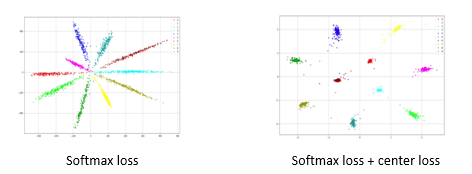# A-Softmax Loss

$W_1^Tx+b = X_2^Tx+b \to (W_1^T - W_2^T)x - (b_1 - b_2) = 0$

$||W_1||||x||cos(\theta_1) + b_1 = ||W_2||||x||cos(\theta_2) + b_2$

$L_i=\frac{1}{N} ∑_i-\log(\frac{ e^{∥x_i∥\cos⁡(mθ_(y_i ),i) }}{e^{∥xi∥\cos(mθyi,i)} + \sum_{j ≠yi}e^{∥xi∥\cos(θj,i)}})$

Softmax，Modified Softmax， A-Softmax作用下的特征分布如图7所示：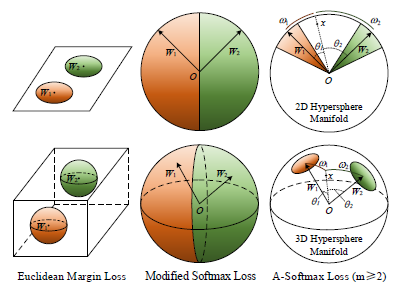Cosine 距离是一个非线性距离，在人脸度量上比线性的欧式距离更有优势，加入超参数m，相当于在原有角度距离的基础上，限制了类内距离，保证类间距离大的同时类内距离小。正是如此，该算法也取得了Megaface竞赛的第一名。

# 参考文献：

 Alex Krizhevsky, Ilya Sutskever, Geoffrey E. Hinton ImageNet Classification with Deep Convolutional Neural Networks NIPS 2012
 http://vis-www.cs.umass.edu/lfw/
 http://www.cbsr.ia.ac.cn/english/CASIA-WebFace-Database.html
 https://www.microsoft.com/en-us/research/project/ms-celeb-1m-challenge-recognizing-one-million-celebrities-real-world/
 Sumit Chopra, Raia Hadsell, Yann LeCun Learning a Similarity Metric Discriminatively, with Application to Face Verification. CVPR 2005
 Florian Schroff, Dmitry Kalenichenko , James Philbin. FaceNet: A Unified Embedding for Face Recognition and Clustering CVPR 2015
 Yandong Wen, Kaipeng Zhang, Zhifeng Li, Yu Qiao. A Discriminative Feature Learning Approach
for Deep Face Recognition ECCV 2016
 Weiyang Liu, Yandong Wen, Zhiding Yu, Ming Li, Bhiksha Raj, Le Song SphereFace: Deep Hypersphere Embedding for Face Recognition CVPR 2017

2016-09-07 14:15:34 qq_36075166 阅读数 1085
• ###### 卷积神经网络与人脸识别系统实战视频教程

卷积神经网络与人脸识别系统实战视频培训课程概况：本教程从零开始讲解人工神经网络，卷积神经网络等基础内容，然后讲解基于python如何构建一个深度学习模型，后基于CNN，利用keras构建网络结构打造一个人脸识别系统。

2558 人正在学习 去看看 王而川2019-06-09 23:30:18 wjh2622075127 阅读数 269
• ###### 卷积神经网络与人脸识别系统实战视频教程

卷积神经网络与人脸识别系统实战视频培训课程概况：本教程从零开始讲解人工神经网络，卷积神经网络等基础内容，然后讲解基于python如何构建一个深度学习模型，后基于CNN，利用keras构建网络结构打造一个人脸识别系统。

2558 人正在学习 去看看 王而川

## 人脸数据获取

code

import cv2

def generate():
)
camera = cv2.VideoCapture(0)
count = 0

while True:
frame = cv2.flip(frame, 1)  # 翻转为正常角度
gray = cv2.cvtColor(frame, cv2.COLOR_BGR2GRAY) #转灰度处理
faces = face_cascade.detectMultiScale(gray, 1.3, 5) # 识别

for (x, y, w, h) in faces:
img = cv2.rectangle( # 画框图
frame, (x, y), (x + w, y + h), (255, 0, 0), 2
)
# cv2.putText(
#     img, 'name', (x, y - 20), cv2.FONT_HERSHEY_SIMPLEX, 1, 255, 2
# )
f = cv2.resize(gray[y: y+h, x: x+w], (200, 200)) # 统一大小
cv2.imwrite('./data/at/name/%s.pgm' % str(count), f) # 将人脸数据写入到pgm文件中
count += 1
if count == 200:
break

cv2.imshow('camera', frame)
if cv2.waitKey(int(1000 / 12)) & 0xff == ord("q"): # 等待ｑ键
break

camera.release()
cv2.destroyAllWindows()
return

if __name__ == '__main__':
generate()


## 处理图片读取到并数组中

import os, sys, re
import cv2
import numpy as np

c = 0
X, y = [], []
dic = {}
for dirname, dirnames, filenames in os.walk(path): # 遍历文件夹下文件
for subdirname in dirnames:
subject_path = os.path.join(dirname, subdirname)
# print("subdirname = ", dirname)
# print("subject_path = ", subject_path)
for filename in os.listdir(subject_path):
if filename[-4:] != '.pgm': # 如果文件不是pgm文件,那么跳过
continue
filepath = os.path.join(subject_path, filename) # 生成完整的文件名
# print(filepath)
# print(np.shape(im))
# 改变大小
if sz is not None:
im = cv2.resize(im, (200, 200)) # 调整图片大小

X.append(np.asarray(im, dtype=np.uint8))
y.append(c)
# print(re.findall(r'./data/at/(.*)', subject_path))
dic[c] = re.findall(r'./data/at/(.*)', subject_path)
c = c + 1
# print("c = ", c)
# print(X)
return [X, y], dic

c = 0
X, y = [], []
dic = {}
for dirname, dirnames, filenames in os.walk(path): # 遍历文件夹下文件
for subdirname in dirnames:
subject_path = os.path.join(dirname, subdirname)
# print("subdirname = ", dirname)
# print("subject_path = ", subject_path)
for filename in os.listdir(subject_path):
if filename[-4:] != '.pgm': # 如果文件不是pgm文件,那么跳过
continue
filepath = os.path.join(subject_path, filename) # 生成完整的文件名
# print(filepath)
ret, im = cv2.threshold(im, 120, 255, cv2.THRESH_BINARY)
if sz is not None:
im = cv2.resize(im, (200, 200)) # 调整图片大小

X.append(np.asarray(im, dtype=np.uint8))
y.append(c)
# print(re.findall(r'./data/at/(.*)', subject_path))
dic[c] = re.findall(r'./data/at/(.*)', subject_path)
c = c + 1
# print("c = ", c)
# print(X)
return [X, y], dic


## 调用opencv内置函数进行人脸识别

model = cv2.face.EigenFaceRecognizer_create() # 这个版本的opencv名字改了,和书上的有点不一样
model = cv2.face.LBPHFaceRecognizer_create(1, 8, 8, 90)
model = cv2.face.FisherFaceRecognizer_create()


code

import cv2
import numpy as np
from PIL import Image, ImageDraw, ImageFont

def cv2ImgAddText(img, text, left, top, textColor=(0, 255, 0), textSize=20):
img = Image.fromarray(cv2.cvtColor(img, cv2.COLOR_BGR2RGB))
draw = ImageDraw.Draw(img)
fontText = ImageFont.truetype(
"SimHei.ttf", textSize, encoding="utf-8")
draw.text((left, top), text, textColor, font=fontText)
return cv2.cvtColor(np.asarray(img), cv2.COLOR_RGB2BGR)

def face_rec(): # 人脸识别

print("label2name:", label2name)
print(np.shape(X))
print("label2name = ", label2name)
print(np.shape(X))
# print("y = ", y)
y = np.asarray(y, dtype=np.int32)

# 调用人脸识别函数生成模型
# model = cv2.face.EigenFaceRecognizer_create() # 这个版本的opencv名字改了,和书上的有点不一样
model = cv2.face.LBPHFaceRecognizer_create(1, 3, 8, 8)
model.train(np.asarray(X), np.asarray(y)) # 训练
camera = cv2.VideoCapture(0)
)
while True:
img = cv2.flip(img, 1)  # 翻转为正常角度
for (x, y, w, h) in faces:
img = cv2.rectangle(img, (x, y), (x+w, y+h), (255, 0, 0), 2)
gray = cv2.cvtColor(img, cv2.COLOR_BGR2GRAY)
roi = gray[x: x+2, y: y+h]
roi = cv2.resize(roi, (200, 200), interpolation=cv2.INTER_LINEAR)
params = model.predict(roi) # 预测
print(params) # 返回 (图片标签, 置信度)
if params >= 0:
name = label2name[params]
else:
name = "未知"
print("检测到%s" % name)
print("label: %s, Confidence: %.2f" % (params, params))
img = cv2ImgAddText(img, name, x, y - 20, (255, 255, 0), 20)
# cv2.putText(img, name, (x, y - 20),
#             cv2.FONT_HERSHEY_SIMPLEX, 1, 255, 2) # putText只能写上ascii中的部分字符, 呵呵
# print(img)

cv2.imshow('camera', img)
if cv2.waitKey(1000 // 12) & 0xff == ord("q"):
break
cv2.destroyAllWindows()

if __name__ == "__main__":
face_rec() # 人脸识别


## knn算法进行人脸识别

knn 实现

import numpy as np
import matplotlib.pyplot as plt

def knn(xtest, data, label, k): # xtest为测试的特征向量，data、label为“训练”数据集，k为设定的阈值
#     print(xtest.shape)
#     print(label.shape)
exp_xtest = np.tile(xtest, (len(label), 1)) - data
sq_diff = exp_xtest**2
sum_diff = sq_diff.sum(axis=1)
distance = sum_diff**0.5
# print(distance)
sort_index = distance.argsort()
classCount = {}
for i in range(k):
one_label = label[sort_index[i]]
classCount[one_label] = classCount.get(one_label, 0) + 1
sortedClassCount = sorted(classCount.items(), key = lambda x:x, reverse=True)
print(distance.sum())
print(classCount)
print(sortedClassCount)
return sortedClassCount

def main():
print(np.shape(X))
X = np.array(X)
Xx = X.reshape(X.shape, 40000)
xdata, label = Xx, y
result = knn(np.array(xdata), xdata, label, 3)
print("result = ", label2name[result])
return result

if __name__ == '__main__':
main()


import cv2
import numpy as np
from PIL import Image, ImageDraw, ImageFont
from knn import knn

def cv2ImgAddText(img, text, left, top, textColor=(0, 255, 0), textSize=20):
img = Image.fromarray(cv2.cvtColor(img, cv2.COLOR_BGR2RGB))
draw = ImageDraw.Draw(img)
fontText = ImageFont.truetype(
"SimHei.ttf", textSize, encoding="utf-8")
draw.text((left, top), text, textColor, font=fontText)
return cv2.cvtColor(np.asarray(img), cv2.COLOR_RGB2BGR)

def face_rec(): # 人脸识别

X = np.array(X)
Xx = X.reshape(X.shape, 40000)
xdata, label = Xx, y

y = np.asarray(y, dtype=np.int32)
)
videoCapture = cv2.VideoCapture(0)
while True:
img = cv2.flip(img, 1)
for (x, y, w, h) in faces:
img = cv2.rectangle(img, (x, y), (x+w, y+h), (255, 0, 0), 2)
gray = cv2.cvtColor(img, cv2.COLOR_BGR2GRAY)
roi = gray[x: x+2, y: y+h]
roi = cv2.resize(roi, (200, 200), interpolation=cv2.INTER_LINEAR)
roi = np.array(roi).reshape(40000, )
result = knn(roi, xdata, label, 3)
print(result) # 返回结果的标签
name = label2name[result]
print("检测到%s" % name)
img = cv2ImgAddText(img, name, x, y - 20, (255, 255, 0), 20)
cv2.imshow('camera', img)
if cv2.waitKey(1000 // 12) & 0xff == ord("q"):
break
cv2.destroyAllWindows()

if __name__ == "__main__":
face_rec() # 人脸识别


## 使用Dense层神经网络进行人脸识别

from getData import read_images, read_images_binary
import numpy as np
from tensorflow.keras import models
from tensorflow.keras import layers

def to_onehot(y): # 将标签转化为独热码oen-hot
print("max of y = ", np.max(y))
onehots = np.zeros((len(y), np.max(y) + 1), dtype=np.float)
for i in range(len(y)):
onehots[i][y[i]] = 1.0
print("shape of onehots : ", np.shape(onehots))
return onehots

def get_model_dense(size=(200, 200)): # 设置一个全连接层网络，返回模型，未训练
model = models.Sequential([
layers.Dense(16, activation='relu', input_shape=((40000, ))),
layers.Dense(16, activation='relu'),
layers.Dense(16, activation='relu'),
layers.Dense(4, activation='softmax')
])
model.compile(
optimizer='rmsprop',
loss='categorical_crossentropy',
metrics=['accuracy']
)
return model

def k_fold_validation(train_data, train_targets):
k = 4
num_val_samples = len(train_data) // k
num_epochs = 5
all_scores = []

for i in range(k):
print('processing fole # ', i)
val_data = train_data[i * num_val_samples: (i + 1) * num_val_samples]
val_targets = train_targets[i * num_val_samples: (i + 1) * num_val_samples]

partial_train_data = np.concatenate(
[train_data[:i * num_val_samples],
train_data[(i + 1) * num_val_samples:]],
axis=0
)
partial_train_targets = np.concatenate(
[train_targets[:i * num_val_samples],
train_targets[(i + 1) * num_val_samples:]],
axis = 0
)
model = get_model_dense()
model.fit(
partial_train_data,
partial_train_targets,
epochs=num_epochs,
batch_size=3
)
'''
model5开始，对图片进行了阈值为１２０的图片二值化处理
model6调整了参数（防止过拟合），从６４调成１６
model7加了一层
'''
model.save('dense_model_7.h5')
val_mse, val_mae = model.evaluate(val_data, val_targets)
all_scores.append(val_mae)

def main():
[X, y], label2name = read_images_binary('./data/at/') # 调用人脸数据
X = np.array(X).reshape(len(X), 40000) / 255
y = to_onehot(np.array(y))
index = np.arange(len(X))
np.random.shuffle(index) # 生成打乱的索引
print("index = ", index)
X = X[index] # 得到打乱的数据
y = y[index]
print(X)
print(y)
print(X.shape)
print(y.shape)
k_fold_validation(X, y)

if __name__ == "__main__":
main()


import cv2
import numpy as np
from PIL import Image, ImageDraw, ImageFont
from knn import knn
from tensorflow.keras import models

def cv2ImgAddText(img, text, left, top, textColor=(0, 255, 0), textSize=20):
img = Image.fromarray(cv2.cvtColor(img, cv2.COLOR_BGR2RGB))
draw = ImageDraw.Draw(img)
fontText = ImageFont.truetype(
"SimHei.ttf", textSize, encoding="utf-8")
draw.text((left, top), text, textColor, font=fontText)
return cv2.cvtColor(np.asarray(img), cv2.COLOR_RGB2BGR)

def face_rec(): # 人脸识别

print("label2name: ", label2name)
X = np.array(X)
Xx = X.reshape(X.shape, 40000)
xdata, label = Xx, y

y = np.asarray(y, dtype=np.int32)
)
videoCapture = cv2.VideoCapture(0)

while True:
img = cv2.flip(img, 1)
for (x, y, w, h) in faces:
img = cv2.rectangle(img, (x, y), (x+w, y+h), (255, 0, 0), 2)
gray = cv2.cvtColor(img, cv2.COLOR_BGR2GRAY)
roi = gray[x: x+2, y: y+h]
roi = cv2.resize(roi, (200, 200), interpolation=cv2.INTER_LINEAR)
roi = np.array(roi).reshape(40000, )
prediction = model.predict(np.array([roi]))
# print(np.shape(roi))
print("dnn predict result :", prediction)
index = np.argmax(prediction) # 最大值所在的索引
name = label2name[index]
print("检测到%s" % name)
img = cv2ImgAddText(img, name, x, y - 20, (255, 255, 0), 20)
cv2.imshow('camera', img)
if cv2.waitKey(1000 // 12) & 0xff == ord("q"):
break
cv2.destroyAllWindows()

if __name__ == "__main__":
face_rec() # 人脸识别


## 一些小知识点

### opencv putText无法写中文

putText()不能直接写上中文，那就用PIL库曲线救国了。下面是一个demo。

#coding=utf-8
#中文乱码处理

import cv2
import numpy
from PIL import Image, ImageDraw, ImageFont

def cv2ImgAddText(img, text, left, top, textColor=(0, 255, 0), textSize=20):
img = Image.fromarray(cv2.cvtColor(img, cv2.COLOR_BGR2RGB))
draw = ImageDraw.Draw(img)
fontText = ImageFont.truetype(
"SimHei.ttf", textSize, encoding="utf-8")
draw.text((left, top), text, textColor, font=fontText)
return cv2.cvtColor(numpy.asarray(img), cv2.COLOR_RGB2BGR)

img = cv2ImgAddText(img, "你好世界", 140, 60, (255, 255, 0), 20)
cv2.imshow('image', img)
cv2.waitKey(0)
cv2.destroyAllWindows()


### 二值化图片

import cv2
import numpy as np

# img = cv2.cvtColor(img, cv2.COLOR_BGR2GRAY)
cv2.imwrite('test.jpg', cv2.Canny(img, 50, 120))
ret, img = cv2.threshold(img, 110, 255, cv2.THRESH_BINARY)
cv2.imshow('binary', img)
cv2.waitKey()
cv2.destroyAllWindows()


### 路径目录测试

import os

path = './data'
for dirname, dirnames, filenames in os.walk(path):
for subdirname in dirnames:
subject_path = os.path.join(dirname, subdirname)
# print(subject_path)
for filename in os.listdir(subject_path):
if filename[-4:] == '.pgm':
filepath = os.path.join(subject_path, filename)
print(filepath)


### np.random.shuffle() 测试

np.random.shuffle() 能够将某一迭代对象进行打乱。

import numpy as np

a = range(0, 10)
print(a)
print(type(a))
print(np.array(a))
index = np.array(a)
np.random.shuffle(index)
print(index)

index = np.arange(7)
print(index)


### keras保存和恢复模型

from tensorflow.keras import models
model.save('dense_model_7.h5')
model = models.load_model('dense_model_6.h5')

2019-05-09 09:09:51 whale52hertz 阅读数 111
• ###### 卷积神经网络与人脸识别系统实战视频教程

卷积神经网络与人脸识别系统实战视频培训课程概况：本教程从零开始讲解人工神经网络，卷积神经网络等基础内容，然后讲解基于python如何构建一个深度学习模型，后基于CNN，利用keras构建网络结构打造一个人脸识别系统。

2558 人正在学习 去看看 王而川

[视频]使用深度学习技术的人脸识别服务器软件

### 松下的深度学习人脸识别软件具有以下特点:

1.精度高:

-由NIST(IJB-A face challenge，是美国最权威的研究机构之一)评估的世界上精度最高的人脸识别引擎；

– iA功能和最佳拍摄图像最大限度的提高人脸识别引擎性能和识别精度。

2.系统成本降低:只发送“最佳拍摄”图像，以减少服务器负载和网络负载。

3.系统可扩展性:

-最多注册30,000张人脸；

-用i-PRO监控系统进行集成管理:通过相同的GUI实现人脸识别系统和监控系统的集成管理。[发展背景]

[产品特点]

1.精度高：
– 由NIST评估的顶级人脸识别引擎（IJB-A面临的挑战）；

– 新软件中使用的深度学习技术与新加坡国立大学联合开发，与传统系统相比，可将人脸识别性能提高达500％；

– 一种结合深度学习的独特算法，与一种抑制错误的相似度计算方法相结合，可以在常规人脸识别技术难以实现的情况下进行识别，例如当脸部倾斜（脸部角度为向左或向右45度、向上或向下30度），由于太阳镜或口罩隐藏部分脸部，或因衰老而改变；

– iA功能和最佳拍摄图像可最大限度地提高人脸识别引擎的性能并提供高识别精度；

– iA功能使相机能够自动检测场景并相应地自动优化设置，以提高视频图像的可检测性。相机会自动检测视频中的移动物体，移动速度，脸部和光线强度（白天/夜晚/头灯），并实时优化设置并获得更佳效果主题的视频；

– 此软件附带的最佳拍摄许可证密钥可以安装在Panasonic i-PRO EXTREME系列网络摄像机上，以便从摄像机前拍摄的多张人脸图像中自动选择适合人脸识别的图像，并只将这些选定的图像发送到服务器。这使得高质量的图像能够在无需加载服务器负载的情况下被发送。

2.系统成本降低：
– 只发送最佳拍摄图像以减少服务器负载和网络负载；

– 使用传统的人脸识别系统时，所有捕获的图像都会发送到服务器，由服务器执行人脸检测和人脸识别，从而将数据处理的负担集中在服务器上。由于发送所有图像所需的大带宽以及保存这些图像所需的高容量硬盘空间，系统也往往是大规模的。

– 将本产品与包含iA功能的相机结合使用的松下系统允许相机检测人脸，并且只有包含脸部的最佳拍摄图像才能发送到服务器，从而显着降低网络负载并消除大型化带宽。使用最佳镜头图像在服务器上执行人脸识别可降低服务器负载和硬盘驱动器容量要求，并允许将多达20 * 5个网络摄像头连接到单台服务器。

– 与传统系统不同，使用该Panasonic系统，不再需要将数据密集型高分辨率图像发送到服务器。该系统可以提供高精度的人脸识别，同时显着降低网络流量，传输成本和网络建设成本。

– 使用连接10台或更多网络摄像机的松下系统时，与不使用最佳拍摄功能的传统系统相比，其成本可降低约40％至50％。

3.系统可扩展性：
– 最多注册30,000张人脸；

– 使用标准人脸识别服务器软件（WV-ASF950），可以对最多10,000张人脸进行简单的批量注册。人脸注册扩展套件（WV-ASFE951W）可在大型设施中注册多达30,000张人脸。

– 使用i-PRO监控系统进行集成管理，通过使用Panasonic i-PRO监视系统的WV-ASM300或WV-ASE231W客户端软件进行集成管理，可以在同一GUI中执行录制视频的人脸检测，人脸识别和跟踪。这消除了传统人脸识别系统所需的专用操作和管理，使得工作可以集中。

Deep Photo Styletransfer的一种纯Tensorflow实现，教你如何转换图片风格

DeepMind认为：神经科学与人工智能的联手已无法阻挡！

2018-08-24 21:39:35 fendouaini 阅读数 1796
• ###### 卷积神经网络与人脸识别系统实战视频教程

卷积神经网络与人脸识别系统实战视频培训课程概况：本教程从零开始讲解人工神经网络，卷积神经网络等基础内容，然后讲解基于python如何构建一个深度学习模型，后基于CNN，利用keras构建网络结构打造一个人脸识别系统。

2558 人正在学习 去看看 王而川

【磐创AI导读】本文是深度学习之视频人脸识别系列的第二篇文章，介绍人脸检测与对齐的相关算法。欢迎大家关注我们的公众号：磐创AI。

1. 问题描述：

1. 深度学习相关算法：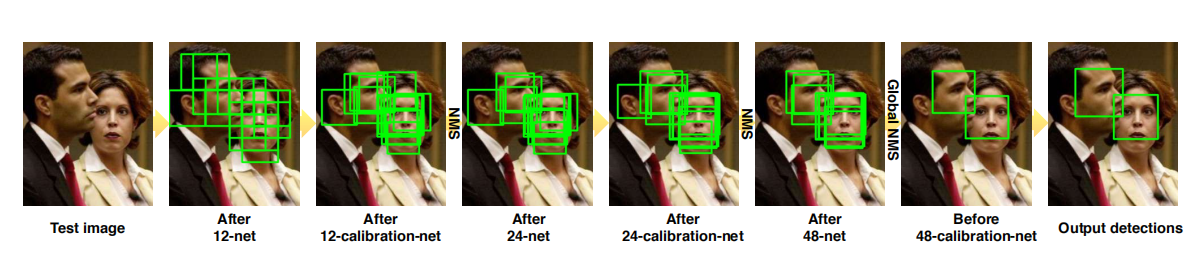12-net，24-net和48-net的网络结构如下图所示：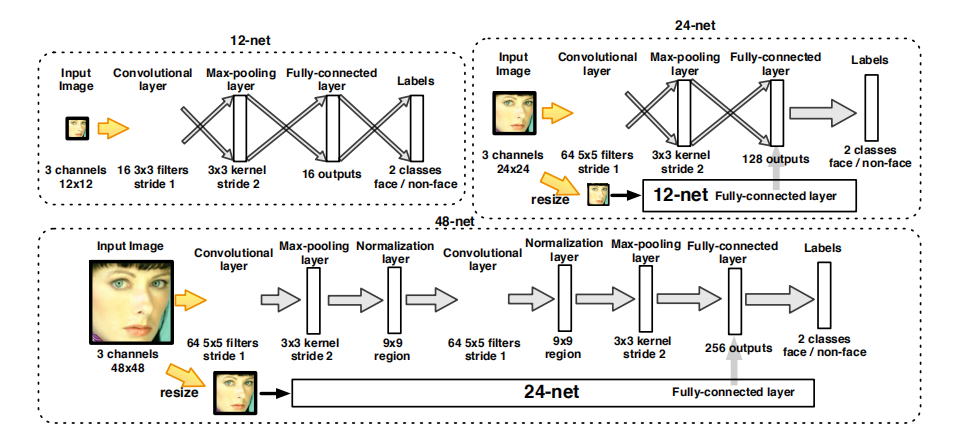13-12-calibration-net，24-calibration-net，48-calibration-net的结构如下图所示：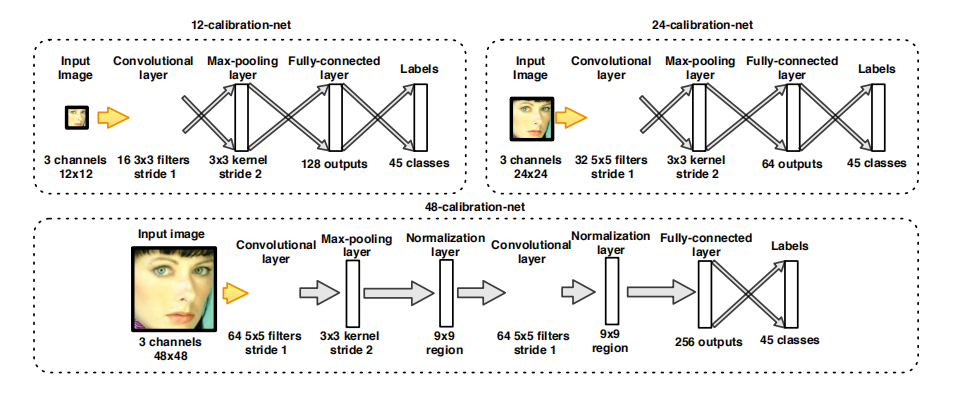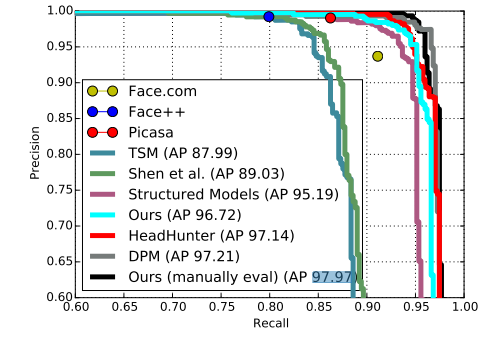（2）Faceness-Net

Faceness-Net源于论文A convolutional neural network cascade for face detection【3】，该算法基于DCNN网络【5】的人脸局部特征分类器，算法首先进行人脸局部特征的检测，使用多个基于DCNN网络的facial parts分类器对人脸进行评估，然后根据每个部件的得分进行规则分析得到Proposal的人脸区域，然后从局部到整体得到人脸候选区域，再对人脸候选区域进行人脸识别和矩形框坐标回归，该过程分为两个步骤。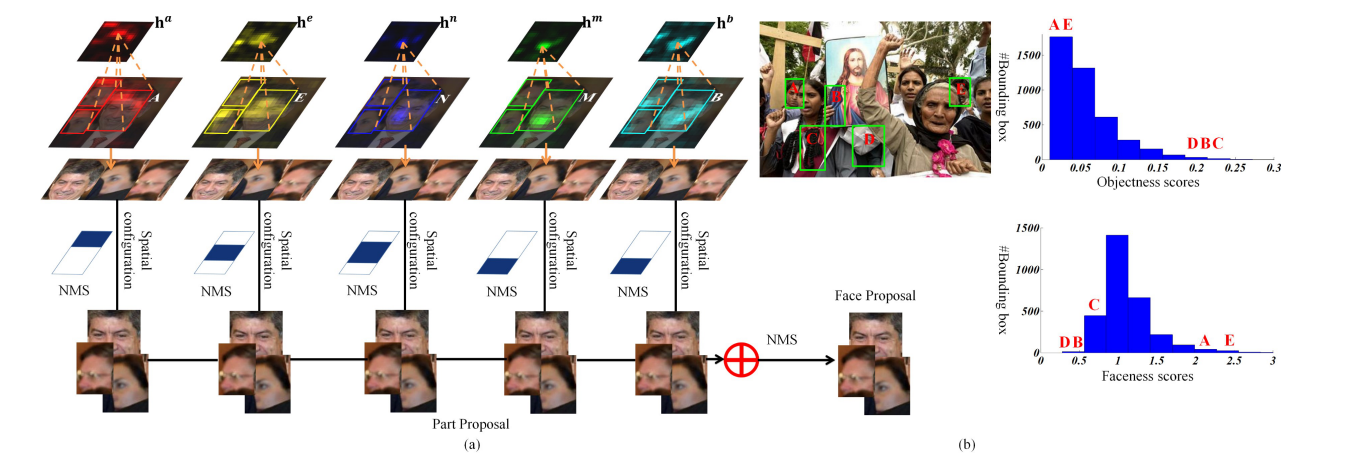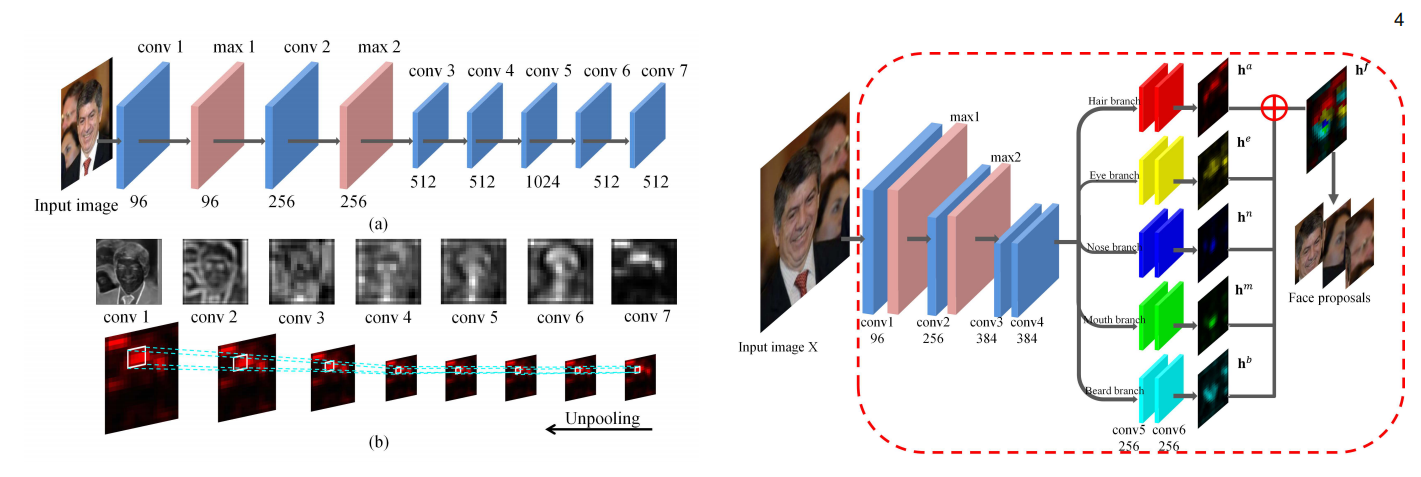Faceness从脸部特征的角度来解决人脸检测中的遮挡和姿态角度问题，其整体性能在当时是非常好的，在AFW数据集上准确率可以达到98.05%。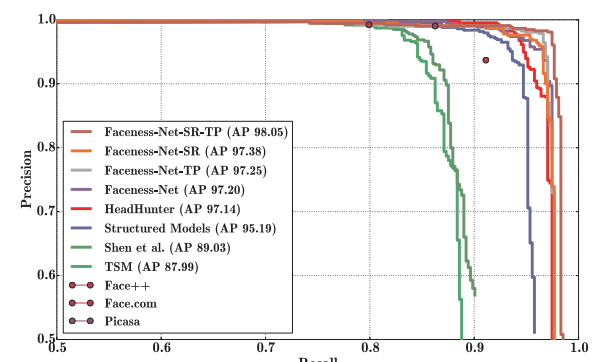（3）MTCNN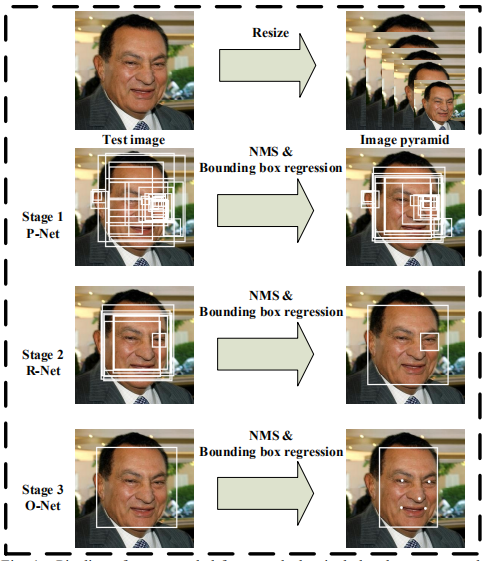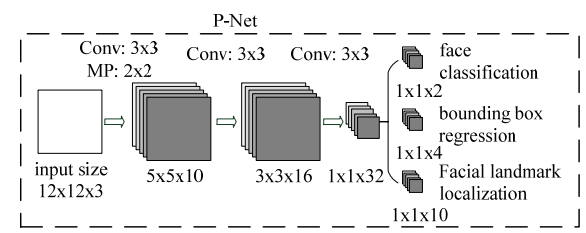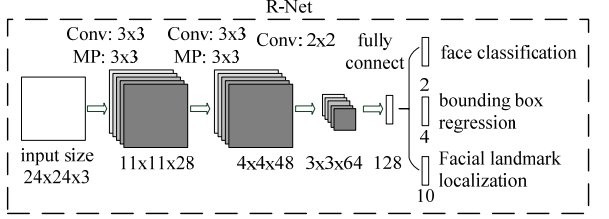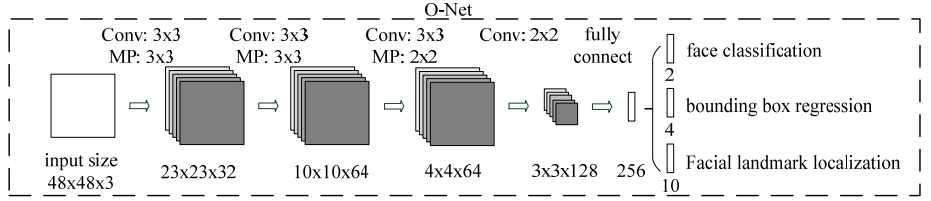MTCNN通过三级的级联卷积神经网络对任务进行从粗到细的处理，还提出在线困难样本生成策略（online hard sample mining ）可以进一步提升性能。兼并了速度与准确率，速度在GPU上可以达到99FPS，在 FDDB数据集上可以达到95.04准确率，具体如下图所示：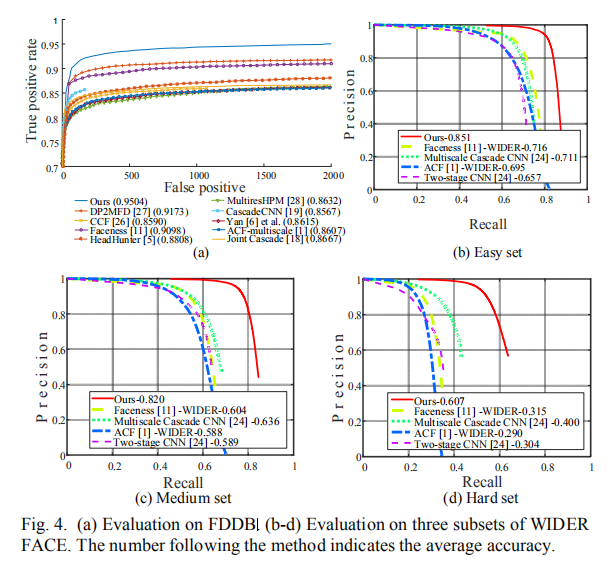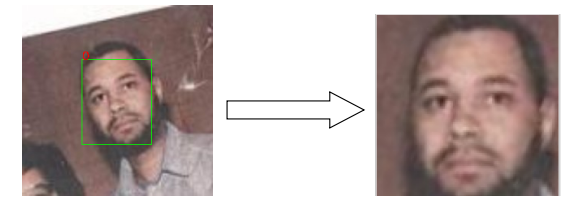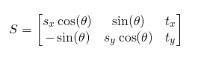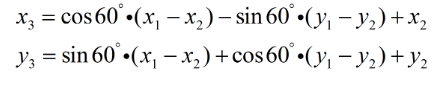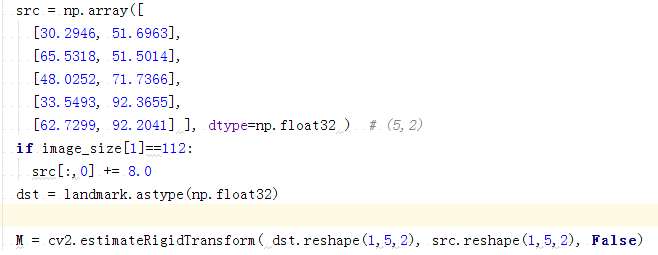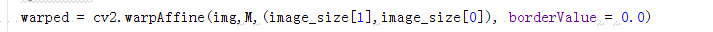img为输入图像;

warped为变换后图像，类型与src一致;

M为变换矩阵，需要通过其它函数获得，当然也可以手动输入;

Image_size为输出图像的大小;

【1】 S.Z.Li, L.Zhu, Z.Q.Zhang, A.Blake, H.J.Zhang, H.Y.Shum. Statistical learning of multi-view face detection. In: Proceedings of the 7-th European Conference on Computer Vision. Copenhagen, Denmark: Springer, 2002.67-81.

【2】Li H, Lin Z, Shen X, et al. A convolutional neural network cascade for face detection[C]//Proceedings of the IEEE Conference on Computer Vision and Pattern Recognition. 2015: 5325-5334.

【3】Yang S, Luo P, Loy C C, et al. Faceness-Net: Face detection through deep facial part responses[J]. IEEE transactions on pattern analysis and machine intelligence, 2017.

【4】Yang S, Luo P, Loy C C, et al. From facial parts responses to face detection: A deep learning approach[C]//Proceedings of the IEEE International Conference on Computer Vision. 2015: 3676-3684.

【5】Sun Y, Wang X, Tang X. Deep convolutional network cascade for facial point detection[C]//Proceedings of the IEEE conference on computer vision and pattern recognition. 2013: 3476-3483.

【6】Zhang K, Zhang Z, Li Z, et al. Joint face detection and alignment using multitask cascaded convolutional networks[J]. IEEE Signal Processing Letters, 2016, 23(10): 1499-1503.